# ISEE Upper Level Math : How to find the area of a sector

## Example Questions

### Example Question #1 : How To Find The Area Of A Sector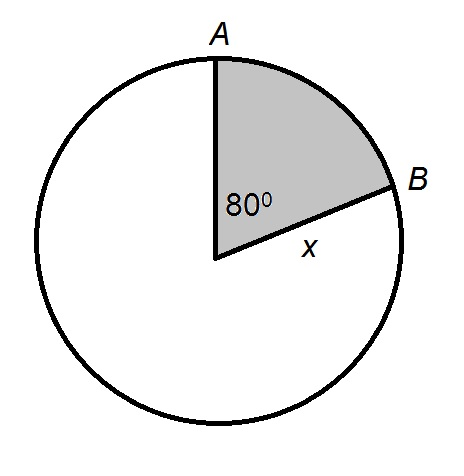Give the area of the white region of the above circle if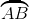has length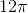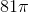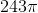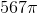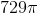Explanation:

If we letbe the circumference of the circle, then the length ofis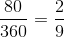of the circumference, so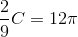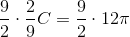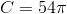The radius is the circumference divided by: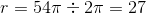Use the formula to find the area of the entire circle: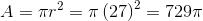The area of the white region is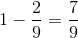of that of the circle, or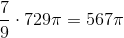### Example Question #71 : CirclesThe circumference of the above circle is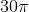. Give the area of the shaded region.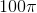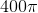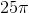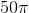Explanation:

The radius of a circle is found by dividing the circumference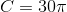by: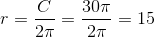The area of the entire circle can be found by substituting forin the formula: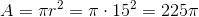.

The area of the shaded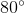sector is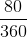of the total area: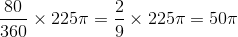### Example Question #3 : How To Find The Area Of A Sector

While visiting a history museum, you see a radar display which consists of a circular screen with a highlighted wedge with an angle of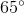. If the screen has a radius of 4 inches, what is the area of the highlighted wedge?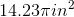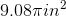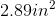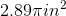Explanation:

While visiting a history museum, you see a radar display which consists of a circular screen with a highlighted wedge with an angle of. If the screen has a radius of 4 inches, what is the area of the highlighted wedge?

To begin, let's recall our formula for area of a sector.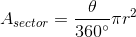Now, we have theta and r, so we just need to plug them in and simplify!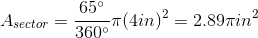So our answer is